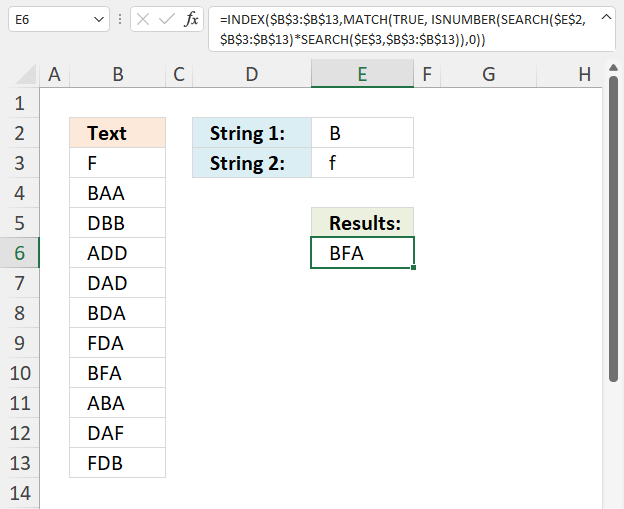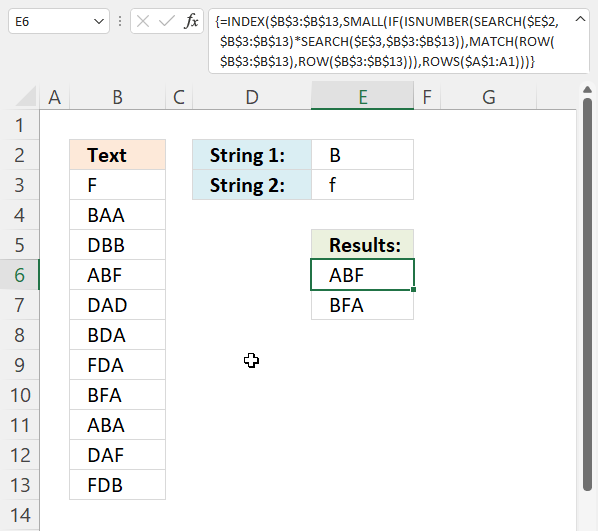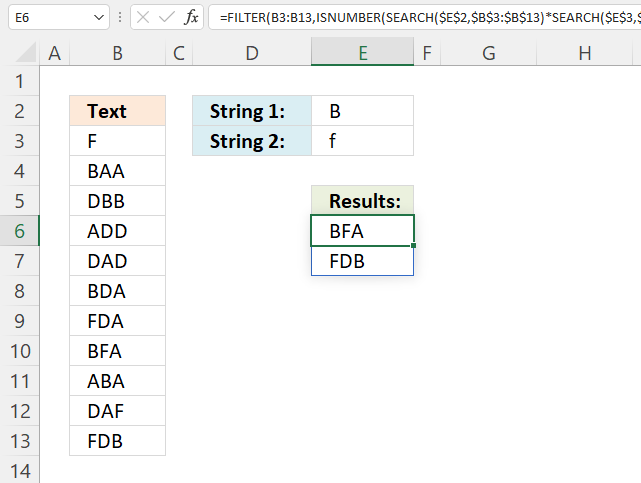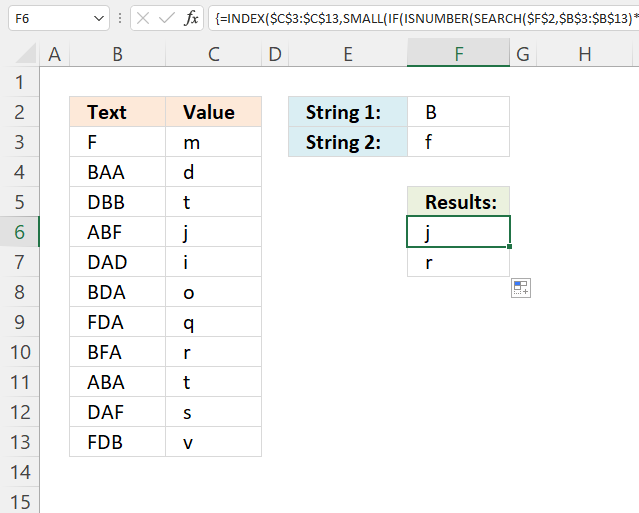Author: Oscar Cronquist Article last updated on November 30, 2021This article demonstrates formulas that let you perform partial matches based on multiple strings and return those strings if all match the same cell. This is AND logic meaning all conditions must be met.

## 1. Partial match for multiple strings - AND logic - returns the first matchThis regular formula returns the first cell that contains both strings from cell range B3:B13, this is not a case-sensitive match.

Formula in cell E6:

=INDEX(\$B\$3:\$B\$13, MATCH(TRUE, ISNUMBER(SEARCH(\$E\$2, \$B\$3:\$B\$13)*SEARCH(\$E\$3, \$B\$3:\$B\$13)), 0))

### 1.1 Explaining formula

#### Step 1 - Partial match based on the first condition

The SEARCH function returns a number representing the position of character at which a specific text string is found reading left to right. It is not a case-sensitive search.

SEARCH(\$E\$2, \$B\$3:\$B\$13)

becomes

and returns

{#VALUE!; 1; 2; #VALUE!; #VALUE!; 1; #VALUE!; 1; 2; #VALUE!; 3}.

Notice the error values, this happens when the SEARCH function can't find the string.

#### Step 2 - Partial match based on second condition

SEARCH(\$E\$3, \$B\$3:\$B\$13)

becomes

and returns

{1; #VALUE!; #VALUE!; #VALUE!; #VALUE!; #VALUE!; 1; 2; #VALUE!; 3; 1}.

#### Step 3 - Multiply arrays AND logic

We need to find cells that contain both strings, we can identify those cells by multiplying the arrays. The result will be a number if both strings are found in the same cell.

Here is the logic behind the calculation:

#VALUE! * number = #VALUE!
number * #VALUE! = #VALUE!
number * number = number

SEARCH(\$E\$2, \$B\$3:\$B\$13)*SEARCH(\$E\$3, \$B\$3:\$B\$13)

becomes

{#VALUE!; 1; 2; #VALUE!; #VALUE!; 1; #VALUE!; 1; 2; #VALUE!; 3}*{1; #VALUE!; #VALUE!; #VALUE!; #VALUE!; #VALUE!; 1; 2; #VALUE!; 3; 1}

and returns

{#VALUE!; #VALUE!; #VALUE!; #VALUE!; #VALUE!; #VALUE!; #VALUE!; 2; #VALUE!; #VALUE!; 3}.

#### Step 4 - Identify numbers

The ISNUMBER function returns TRUE if value is a number and FALSE if not, this works also with error values meaning #VALUE! returns FALSE.

ISNUMBER(value)

ISNUMBER(SEARCH(\$E\$2, \$B\$3:\$B\$13)*SEARCH(\$E\$3, \$B\$3:\$B\$13))

becomes

ISNUMBER({#VALUE!; #VALUE!; #VALUE!; #VALUE!; #VALUE!; #VALUE!; #VALUE!; 2; #VALUE!; #VALUE!; 3})

and returns

{FALSE; FALSE; FALSE; FALSE; FALSE; FALSE; FALSE; TRUE; FALSE; FALSE; TRUE}.

#### Step 5 - Find position of first TRUE in array

The MATCH function returns the relative position of an item in an array or cell reference that matches a specified value in a specific order.

MATCH(lookup_value, lookup_array, [match_type])

MATCH(TRUE, ISNUMBER(SEARCH(\$E\$2, \$B\$3:\$B\$13)*SEARCH(\$E\$3, \$B\$3:\$B\$13)), 0)

becomes

MATCH(TRUE, {FALSE; FALSE; FALSE; FALSE; FALSE; FALSE; FALSE; TRUE; FALSE; FALSE; TRUE}, 0)

and returns 8.

#### Step 6 - Return value from cell range B3:B13

The INDEX function returns a value from a cell range, you specify which value based on a row and column number.

INDEX(\$B\$3:\$B\$13, MATCH(TRUE, ISNUMBER(SEARCH(\$E\$2, \$B\$3:\$B\$13)*SEARCH(\$E\$3, \$B\$3:\$B\$13)), 0))

becomes

INDEX(\$B\$3:\$B\$13, 8)

becomes

INDEX{"F"; "BAA"; "DBB"; "ADD"; "DAD"; "BDA"; "FDA"; "BFA"; "ABA"; "DAF"; "FDB"}, 8)

and returns "BFA".

## 2. Partial match for multiple strings - AND logic - returns all valuesThis regular formula returns the all cell values that contain both strings from cell range B3:B13, this is not a case-sensitive match.

Formula in cell E6:

=INDEX(\$B\$3:\$B\$13, SMALL(IF(ISNUMBER(SEARCH(\$E\$2, \$B\$3:\$B\$13)*SEARCH(\$E\$3, \$B\$3:\$B\$13)), MATCH(ROW(\$B\$3:\$B\$13), ROW(\$B\$3:\$B\$13))), ROWS(\$A\$1:A1)))

### 1.1 Explaining formula

#### Step 1 - Partial match based on the first condition

The SEARCH function returns a number representing the position of character at which a specific text string is found reading left to right. It is not a case-sensitive search.

SEARCH(\$E\$2, \$B\$3:\$B\$13)

becomes

SEARCH("B",{"F"; "BAA"; "DBB"; "ABF"; "DAD"; "BDA"; "FDA"; "BFA"; "ABA"; "DAF"; "FDB"})

and returns

{#VALUE!; 1; 2; 2; #VALUE!; 1; #VALUE!; 1; 2; #VALUE!; 3}.

Notice the error values, they appear if the string is not found at all.

#### Step 2 - Partial match based on the second condition

SEARCH(\$E\$3, \$B\$3:\$B\$13)

becomes

SEARCH("f",{"F"; "BAA"; "DBB"; "ABF"; "DAD"; "BDA"; "FDA"; "BFA"; "ABA"; "DAF"; "FDB"})

and returns

{1; #VALUE!; #VALUE!; 3; #VALUE!; #VALUE!; 1; 2; #VALUE!; 3; 1}.

#### Step 3 - Multiply arrays

We need to find cells that contain both strings, we can identify those cells by multiplying the arrays. The result will be a number if both strings are found in the same cell.

Here is the logic behind the calculation:

#VALUE! * number = #VALUE!
number * #VALUE! = #VALUE!
number * number = number

SEARCH(\$E\$2, \$B\$3:\$B\$13)*SEARCH(\$E\$3, \$B\$3:\$B\$13)

becomes

{#VALUE!; 1; 2; 2; #VALUE!; 1; #VALUE!; 1; 2; #VALUE!; 3}*{1; #VALUE!; #VALUE!; 3; #VALUE!; #VALUE!; 1; 2; #VALUE!; 3; 1}

and returns

{#VALUE!; #VALUE!; #VALUE!; 6; #VALUE!; #VALUE!; #VALUE!; 2; #VALUE!; #VALUE!; 3}.

#### Step 4 - Identify numbers

The ISNUMBER function returns TRUE if value is a number and FALSE if not, this works also with error values meaning #VALUE! returns FALSE.

ISNUMBER(value)

ISNUMBER(SEARCH(\$E\$2, \$B\$3:\$B\$13)*SEARCH(\$E\$3, \$B\$3:\$B\$13))

becomes

ISNUMBER({#VALUE!; #VALUE!; #VALUE!; 6; #VALUE!; #VALUE!; #VALUE!; 2; #VALUE!; #VALUE!; 3})

and returns

{FALSE; FALSE; FALSE; TRUE; FALSE; FALSE; FALSE; TRUE; FALSE; FALSE; TRUE}.

#### Step 5 - Replace True with row number

The IF function returns one value if the logical test is TRUE and another value if the logical test is FALSE.

IF(logical_test, [value_if_true], [value_if_false])

We want to substitute True with the corresponding row number in order to get the correct value in step 7.

IF(ISNUMBER(SEARCH(\$E\$2, \$B\$3:\$B\$13)*SEARCH(\$E\$3, \$B\$3:\$B\$13)), MATCH(ROW(\$B\$3:\$B\$13), ROW(\$B\$3:\$B\$13)))

becomes

IF({FALSE; FALSE; FALSE; TRUE; FALSE; FALSE; FALSE; TRUE; FALSE; FALSE; TRUE}, MATCH(ROW(\$B\$3:\$B\$13), ROW(\$B\$3:\$B\$13)))

The ROW function calculates the row number of a cell reference.

ROW(reference)

This works fine with a reference to a cell range as well, the function returns an array of numbers.

ROW(\$B\$3:\$B\$13)

returns

{3; 4; 5; 6; 7; 8; 9; 10; 11; 12; 13}

The MATCH function returns the relative position of an item in an array or cell reference that matches a specified value in a specific order.

MATCH(lookup_value, lookup_array, [match_type])

MATCH(ROW(\$B\$3:\$B\$13), ROW(\$B\$3:\$B\$13))

becomes

MATCH({3; 4; 5; 6; 7; 8; 9; 10; 11; 12; 13}, {3; 4; 5; 6; 7; 8; 9; 10; 11; 12; 13})

and returns

{1; 2; 3; 4; 5; 6; 7; 8; 9; 10; 11}.

IF({FALSE; FALSE; FALSE; TRUE; FALSE; FALSE; FALSE; TRUE; FALSE; FALSE; TRUE}, MATCH(ROW(\$B\$3:\$B\$13), ROW(\$B\$3:\$B\$13)))

becomes

IF({FALSE; FALSE; FALSE; TRUE; FALSE; FALSE; FALSE; TRUE; FALSE; FALSE; TRUE}, {1; 2; 3; 4; 5; 6; 7; 8; 9; 10; 11})

and returns

{FALSE; FALSE; FALSE; 4; FALSE; FALSE; FALSE; 8; FALSE; FALSE; 11}.

#### Step 6 - Get the k-th smallest row number

The SMALL function returns the k-th smallest value from a group of numbers. The first argument is a cell range or array that you want to find the k-th smallest number from. The SMALL function ignores text and boolean values.

SMALL(arrayk)

SMALL(IF(ISNUMBER(SEARCH(\$E\$2, \$B\$3:\$B\$13)*SEARCH(\$E\$3, \$B\$3:\$B\$13)), MATCH(ROW(\$B\$3:\$B\$13), ROW(\$B\$3:\$B\$13))), ROWS(\$A\$1:A1))

becomes

SMALL({FALSE; FALSE; FALSE; 4; FALSE; FALSE; FALSE; 8; FALSE; FALSE; 11}, ROWS(\$A\$1:A1))

The ROWS function returns a number representing the number of rows in a  reference.

ROWS(ref)

ROWS(\$A\$1:A1) contains the following cell reference \$A\$1:A1, it contains both an absolute and relative cell reference making it a growing cell reference. This means that when the formula is copied to cells below the cell reference expands automatically. This will return a larger number for each cell below making the formula get a new row number in each cell.

SMALL({FALSE; FALSE; FALSE; 4; FALSE; FALSE; FALSE; 8; FALSE; FALSE; 11}, ROWS(\$A\$1:A1))

becomes

SMALL({FALSE; FALSE; FALSE; 4; FALSE; FALSE; FALSE; 8; FALSE; FALSE; 11}, 1)

and returns 4.

#### Step 7 - Get value

The INDEX function returns a value from a cell range, you specify which value based on a row and column number.

INDEX(\$B\$3:\$B\$13, SMALL(IF(ISNUMBER(SEARCH(\$E\$2, \$B\$3:\$B\$13)*SEARCH(\$E\$3, \$B\$3:\$B\$13)), MATCH(ROW(\$B\$3:\$B\$13), ROW(\$B\$3:\$B\$13))), ROWS(\$A\$1:A1)))

becomes

INDEX(\$B\$3:\$B\$13, 4)

and returns the value in cell B6 which is "ABF". The fourth cell in \$B\$3:\$B\$13 is cell B6.

## 3. Partial match for multiple strings - AND logic - returns all matches (Excel 365)The following formula extracts values from cell range B3:B13 if both strings are found in a cell, in other words, a partial match for both conditions.

Notice that the formula is much smaller than the previous Excel versions. The new FILTER function is great, it simplifies the formula and makes it easier to understand.

Dynamic array formula in cell E6:

=FILTER(B3:B13, ISNUMBER(SEARCH(\$E\$2, \$B\$3:\$B\$13)*SEARCH(\$E\$3, \$B\$3:\$B\$13)))

### Explaining formula in cell E6

#### Step 1 - Partial match based on the first condition

The SEARCH function returns a number representing the position of character at which a specific text string is found reading left to right. It is not a case-sensitive search.

SEARCH(\$E\$2, \$B\$3:\$B\$13)

becomes

SEARCH("B",{"F"; "BAA"; "DBB"; "ABF"; "DAD"; "BDA"; "FDA"; "BFA"; "ABA"; "DAF"; "FDB"})

and returns

{#VALUE!; 1; 2; 2; #VALUE!; 1; #VALUE!; 1; 2; #VALUE!; 3}.

Notice the error values, they appear if the string is not found at all.

#### Step 2 - Partial match based on the second condition

SEARCH(\$E\$3, \$B\$3:\$B\$13)

becomes

SEARCH("f",{"F"; "BAA"; "DBB"; "ABF"; "DAD"; "BDA"; "FDA"; "BFA"; "ABA"; "DAF"; "FDB"})

and returns

{1; #VALUE!; #VALUE!; 3; #VALUE!; #VALUE!; 1; 2; #VALUE!; 3; 1}.

#### Step 3 - Multiply arrays

We need to find cells that contain both strings, we can identify those cells by multiplying the arrays. The result will be a number if both strings are found in the same cell.

Here is the logic behind the calculation:

#VALUE! * number = #VALUE!
number * #VALUE! = #VALUE!
number * number = number

SEARCH(\$E\$2, \$B\$3:\$B\$13)*SEARCH(\$E\$3, \$B\$3:\$B\$13)

becomes

{#VALUE!; 1; 2; 2; #VALUE!; 1; #VALUE!; 1; 2; #VALUE!; 3}*{1; #VALUE!; #VALUE!; 3; #VALUE!; #VALUE!; 1; 2; #VALUE!; 3; 1}

and returns

{#VALUE!; #VALUE!; #VALUE!; 6; #VALUE!; #VALUE!; #VALUE!; 2; #VALUE!; #VALUE!; 3}.

#### Step 4 - Identify numbers

The ISNUMBER function returns TRUE if value is a number and FALSE if not, this works also with error values meaning #VALUE! returns FALSE.

ISNUMBER(value)

ISNUMBER(SEARCH(\$E\$2, \$B\$3:\$B\$13)*SEARCH(\$E\$3, \$B\$3:\$B\$13))

becomes

ISNUMBER({#VALUE!; #VALUE!; #VALUE!; 6; #VALUE!; #VALUE!; #VALUE!; 2; #VALUE!; #VALUE!; 3})

and returns

{FALSE; FALSE; FALSE; TRUE; FALSE; FALSE; FALSE; TRUE; FALSE; FALSE; TRUE}.

#### Step 5 - Get values

The FILTER function lets you extract values/rows based on a condition or criteria. It is in the Lookup and reference category and is only available to Excel 365 subscribers.

FILTER(B3:B13, ISNUMBER(SEARCH(\$E\$2, \$B\$3:\$B\$13)*SEARCH(\$E\$3, \$B\$3:\$B\$13)))

becomes

FILTER(B3:B13, {FALSE; FALSE; FALSE; TRUE; FALSE; FALSE; FALSE; TRUE; FALSE; FALSE; TRUE})

and returns

{"BFA"; "FDB"}.

## 4. Partial match for multiple strings - AND logic - returns all corresponding valuesThis formula extracts values from cell range C3:C13 if the corresponding cell in B3;B13 contains both strings specified in cell F2 and F3.

The formula is almost identical to the formula in section 2, only the first cell reference is changed.

Array formula in cell F6:

=INDEX(\$C\$3:\$C\$13, SMALL(IF(ISNUMBER(SEARCH(\$F\$2, \$B\$3:\$B\$13)*SEARCH(\$F\$3, \$B\$3:\$B\$13)), MATCH(ROW(\$B\$3:\$B\$13), ROW(\$B\$3:\$B\$13))), ROWS(\$A\$1:A1)))

Read the explanation in section 2 if you want to know more about the formula.